Dr. J's Maths.com
Where the techniques of Maths
are explained in simple terms.

Trigonometric functions - Calculus - Reverse Chain Rule.
Test Yourself 1.

 No logs here. 1.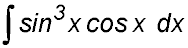Hint.Let u = sin x. 2.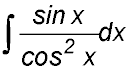Hint.Let u = cos x. 3.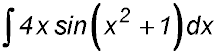Hint.Let u = x2+1. 4.. Hint.Change the tan and sec terms into sin and cos components. Then let u = cos 2x. 5.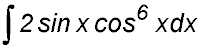. Hint.Let n = cos x. 6.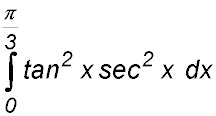Hint.Let m = tan x. Answer.Integral = 3√3. Incorporates logs. 7. Find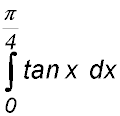Hint.Split the tan ratio into sin over cos. Then let u = cos x. Answer.Integral = (1/2) ln2. 8.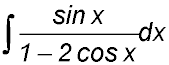Hint.Let u = 1 - 2cos x. 9.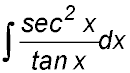Hint.Let u = tan x. 10.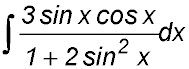Hint.Let u = 1 + 2sin2x. Miscellaneous 11. (i) Show that tan3 x can be written as tanx sec2 x - tan x. (ii) Hence or otherwise show that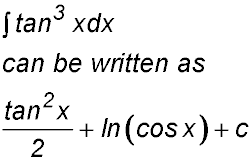Hint.In part (ii), use a reverse chain rule on the two terms separately. 12. (i) Differentiate sin (x2). (ii) Hence or otherwise, find the area bounded by y = xcos (x2), the x-axis and the lines x = 0 and x = 1. (answer to 2 decimal places).Answer.Area = 0.42 u2.